To perform deletion in a circular linked list we need to maintain and store the reference of the last node of the list. because using the reference of the last node the deletion operation can be done in constant time.

so in this tutorial, we are going to learn how to
1. Delete the first node of the list.
2. Delete the only node of the list.
3. Delete the last node of the list.
4. Delete a node at any position in the list.

### Delete the first node of the circular linked list.

let's say we have a list that has four nodes in it.to delete the first node of the list we simply store the second node's reference into the last node's link part.so now the first node is deleted from the linked list.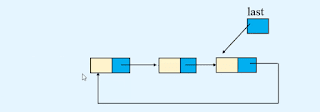### Delete the only node of the circular linked list.

if we have a linked list that has only one node.then to delete this only node we simply store None or Null value into the last variable.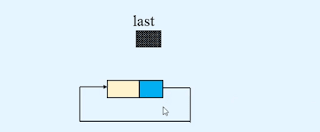so now our list becomes empty.### Delete the last node of the circular linked list.

to delete the last node first we need to find a reference p of the predecessor of the last node.then we store the first node's reference into the linked part of node p.after that, we update the list variable's position. because after deletion of the last node now the node p becomes the last node of the list.so now after performing these operations the last node's is deleted.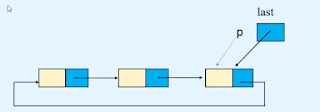### Delete a node at the position in a circular linked list.

to delete a node at position x. first, we need to find the reference to the predecessor of the node. like we want to delete the third node on the list. then we need to find the reference p of the second node.after that, we store the node's reference that comes after the node that we want to delete in the linked part of node p.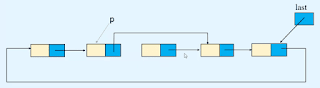so now the third node's deleted from the linked list.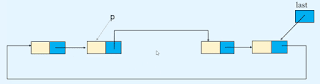#### Python program code for performing deletion in the circular linked list.

```class Node(object):

def __init__(self, value):
self.info = value

def __init__(self):
self.last = None
def display_list(self):

if self.last == None:
print("List is empty\n")
return        p = self.last.link

while True:
print(p.info, " ", end='')
if p == self.last.link:
break        print()

def insert_in_beginning(self, data):
temp = Node(data)

def insert_in_empty_list(self, data):
temp = Node(data)
self.last = temp

def insert_at_end(self,data):
temp = Node(data)
self.last = temp

def create_list(self):
n = int(input("Enter the number of nodes : "))
if n == 0:
return        data = int(input("Enter the element to be inserted : "))
self.insert_in_empty_list(data)

for i in range(n-1):
data = int(input("Enter the element to be inserted : "))
self.insert_at_end(data)

def insert_after(self, data, x):

while True:
if p.info == x:
break            p = p.link
if p == self.last.link:
break
if p == self.last.link and p.info != x:
print(x, " not present in the list")
else:
temp = Node(data)
if p == self.last:
self.last = temp

def delete_first_node(self):
if self.last is None:
return        if self.last.link == self.last:

def delete_last_node(self):
if self.last is None:
return        if self.last.link == self.last:
self.last = None            return
while p.link != self.last:
self.last = p

def delete_node(self,x):

if self.last is None:
return        if self.last.link == self.last and self.last.info == x:
self.last = None            return        if self.last.link.info == x:
return
if p.link.info == x:
break            p = p.link

print(x, " not found in the list")
else:
if self.last.info == x:
self.last = p

#################################################

list.create_list()

while True:
print("1. Display list")
print("2. Insert in empty list")
print("3. Insert a node in the beginning of the list")
print("4. Insert a node at the end of the list")
print("5. Insert a node after a specified node")
print("6. Delete first node")
print("7. Delete last node")
print("8. Delete any node")
print("9. Quite")

option = int(input("Enter your choice : "))

if option == 1:
list.display_list()
elif option == 2:
data = int(input("Enter the element to be inserted : "))
list.insert_in_empty_list(data)
elif option == 3:
data = int(input("Enter the element to be inserted : "))
list.insert_in_beginning(data)
elif option == 4:
data = int(input("Enter the element to be inserted : "))
list.insert_at_end(data)
elif option == 5:
data = int(input("Enter the element to be inserted : "))
x = int(input("Enter the element after which to insert : "))
list.insert_after(data,x)
elif option == 6:
list.delete_first_node()
elif option == 7:
list.delete_last_node()
elif option == 8:
data = int(input("Enter the element to be deleted : "))
list.delete_node(data)
elif option == 9:
break    else:
print("Wrong option")
print()```